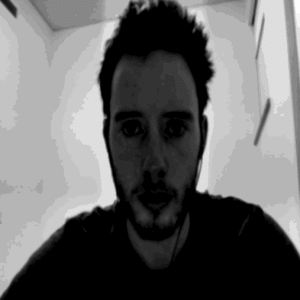# Convolutions

Convolving two functions and amounts to dragging one function over another. Computing the convolution of two functions involves computing the integral of the product of the two functions as one is shifted while the other is stationary. There is a cool theorem (the convolution theorem) that states that the convolution of two functions is equal to their product in Fourier space. I leverage this theorem in my processing code to compute the convolution of the webcam feed with a gaussian. The position of the gaussian can be shifted by moving the cursor across the frame. By switching modes, one can increase the standard deviation of the gaussian, which results in less blurring. I made a GIF that demonstrates this functionality.